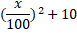# Quiz Discussion

The average of the largest and smallest 3 digits numbers formed by 0, 2 and 4 would be :

Course Name: Quantitative Aptitude

• 1] 312
• 2] 222
• 3] 213
• 4] 303
##### Solution
No Solution Present Yet

#### Top 5 Similar Quiz - Based On AI&ML

Quiz Recommendation System API Link - https://fresherbell-quiz-api.herokuapp.com/fresherbell_quiz_api

# Quiz
1
Discuss

In an exam, the average marks obtained by Jhon in English, Math, Hindi and Drawing were 50. His average mark in Maths, Science, Social Studies and Craft were 70. If the average mark in all seven subjects is 58, his score in Maths was -

• 1] 50
• 2] 52
• 3] 60
• 4] 74
##### Solution
2
Discuss

The average of 6 numbers is 7. The average of three numbers of them is 5. What will be the average of remaining numbers?

• 1] 15
• 2] 30
• 3] 9
• 4] 42
##### Solution
3
Discuss

Sachin Tendulkar has a certain average for 11 innings. In the 12th innings he scores 120 runs and thereby increases his average by 5 runs. His new average is :

• 1] 60
• 2] 62
• 3] 65
• 4] 66
##### Solution
4
Discuss

Ajay working in a Cellular company as a salesman. His monthly salary is Rs. 200. But he gets bonus as per given rule. If he sells sim cards of Rs. X then his bonus will be. In the first quarter of the year his average sale was Rs. 3000 per month. In the next 5 five month his average sale was Rs. 5000 per month and for next four month his average sale was Rs. 8000 per month. What is the average earning per month for the whole year?

• 1] Rs. 3350
• 2] Rs. 3610
• 3] Rs. 3750
• 4] Rs. 3560
• 5] None of these
##### Solution
5
Discuss

Four years ago, the average age of A and B was 18 years. At present the average age of A, B, and C is 24 years. What would be the age of C after 8 years?

• 1] 25 years
• 2] 28 years
• 3] 32 years
• 4] 36 years
##### Solution
6
Discuss

Students of three different classes appeared in common examination. Pass average of 10 students of first class was 70%, pass average of 15 students of second class was 60% and pass average of 25 students of third class was 80% then what will be the pass average of all students of three classes?

• 1] 74%
• 2] 75%
• 3] 69%
• 4] 72%
##### Solution
7
Discuss

A shop of electronic goods is closed on Monday. The average daily sales for remaining six days of a week is Rs. 15,640 and the average sale of Tuesday to Saturday is Rs. 14,124. The sales on Sunday is -

• 1] Rs. 20,188
• 3] Rs. 23,220
• 4] Rs. 21,704
##### Solution
8
Discuss

The average (arithmetic mean) of 330, 360 and 390 is :

• 1]

$${3^{27}} + {3^{57}} + {3^{87}}$$

• 2]

$${3^{29}} + {3^{59}} + {3^{89}}$$

• 3]

$${3^{60}}$$

• 4]

$${3^{177}}$$

##### Solution
9
Discuss

The average score of a class of boys and girls in an examination is A. The ratio of boys and girls in the class is 3 : 1. If the average score of the boys is A + 1, the average score of the girls is-

• 1] A - 1
• 2] A - 3
• 3] A + 1
• 4] A + 3
##### Solution
10
Discuss

The average age of the boys in a class is 16 years and that of the girls is 15 years. The average age for the whole class is-

• 1] 15 years
• 2] 15.5 years
• 3] 16 years
• 4] Cannot be computed with the given information
# Quiz